# RS Aggarwal Solutions for Class 9 Chapter 7: Lines and Angles Exercise 7B

The problems are solved in such a way that they help students understand the topics and improve their problem solving abilities. Practising lots of examples before solving the main exercise makes them familiar with the formulas and methods. The solutions are prepared by experts at BYJU’S with the aim of helping students score well in the board exams. In the CBSE exam pattern each step carries marks, so the answers are prepared with explanations to make it easy for understanding. RS Aggarwal Solutions for Class 9 Maths Chapter 7 Lines and Angles Exercise 7B are provided here.

## RS Aggarwal Solutions for Class 9 Chapter 7: Lines and Angles Exercise 7B Download PDF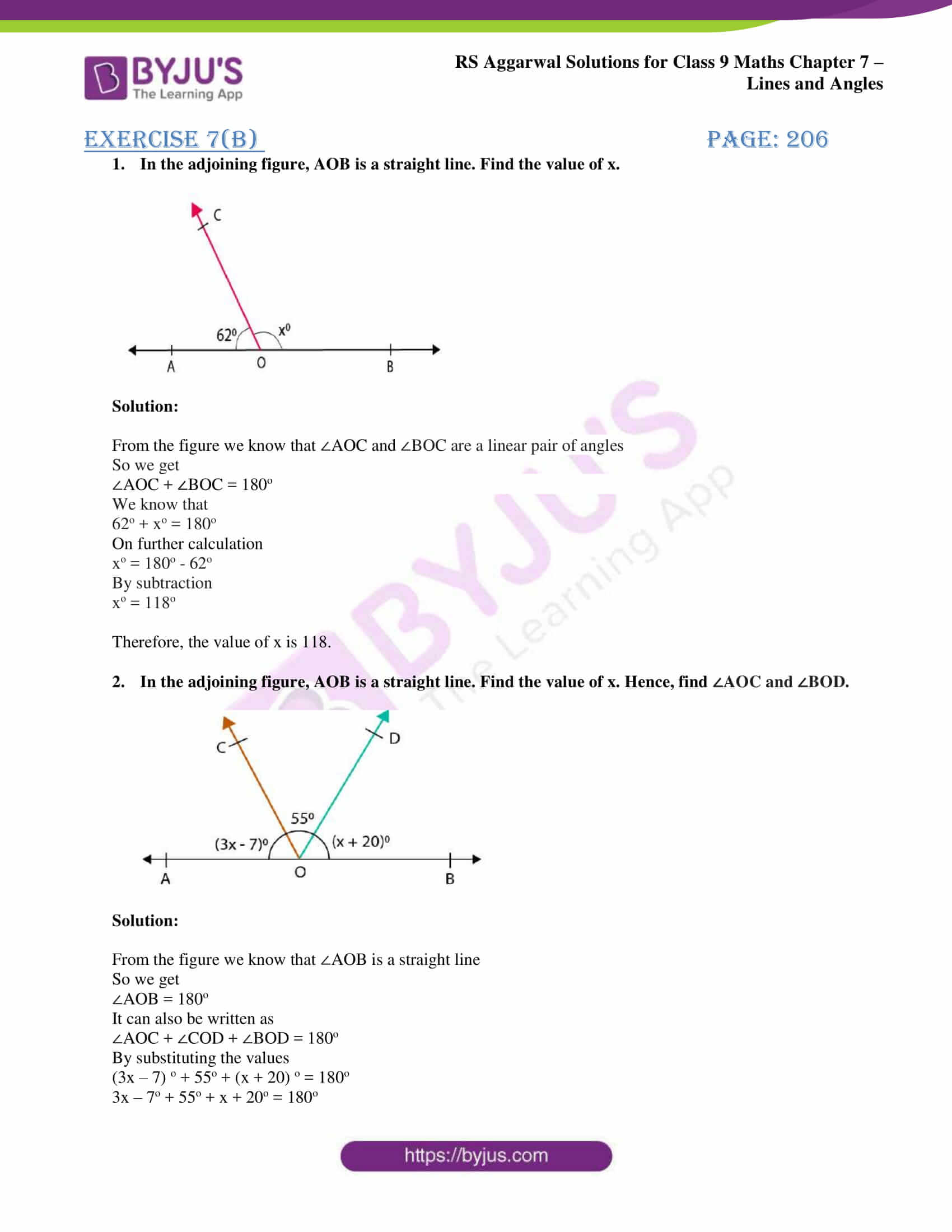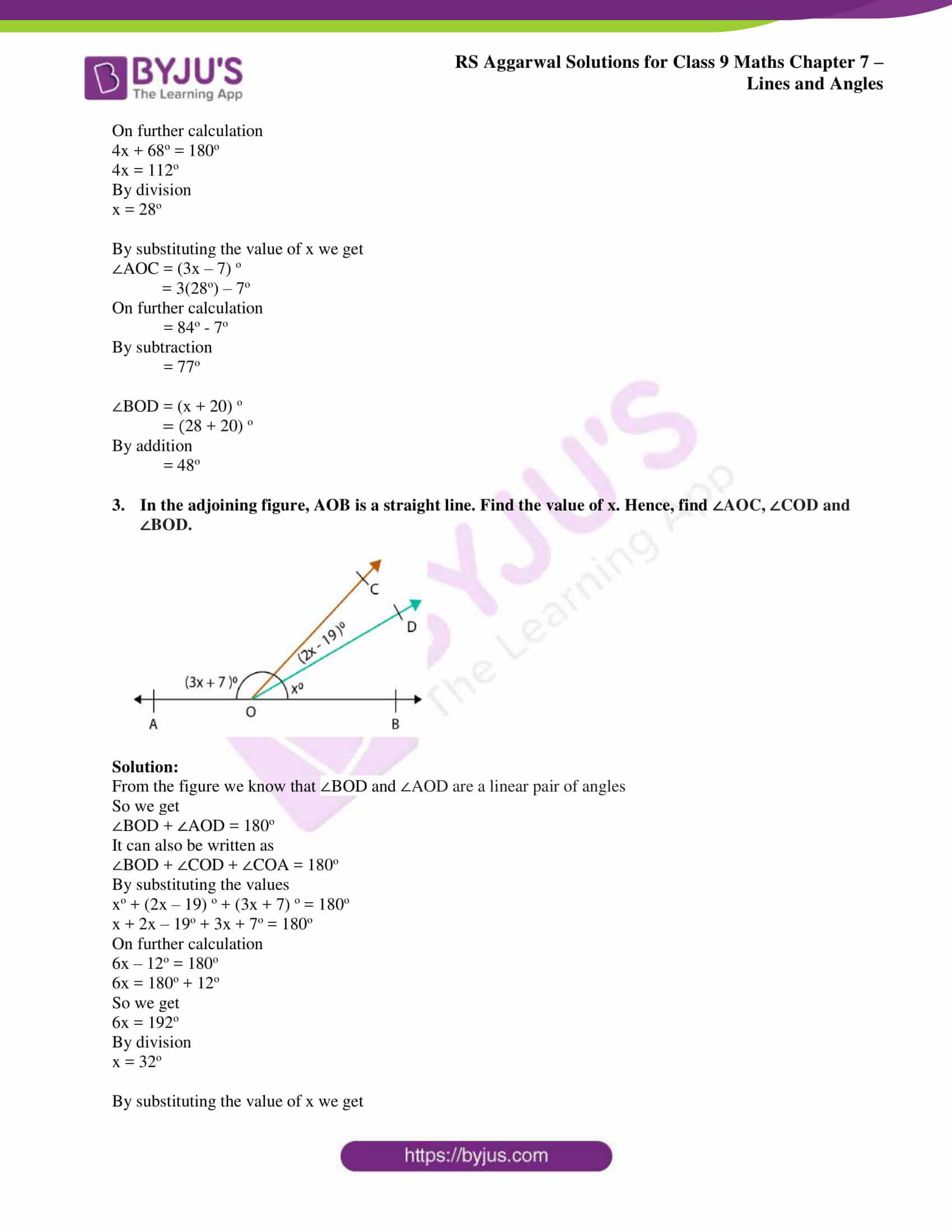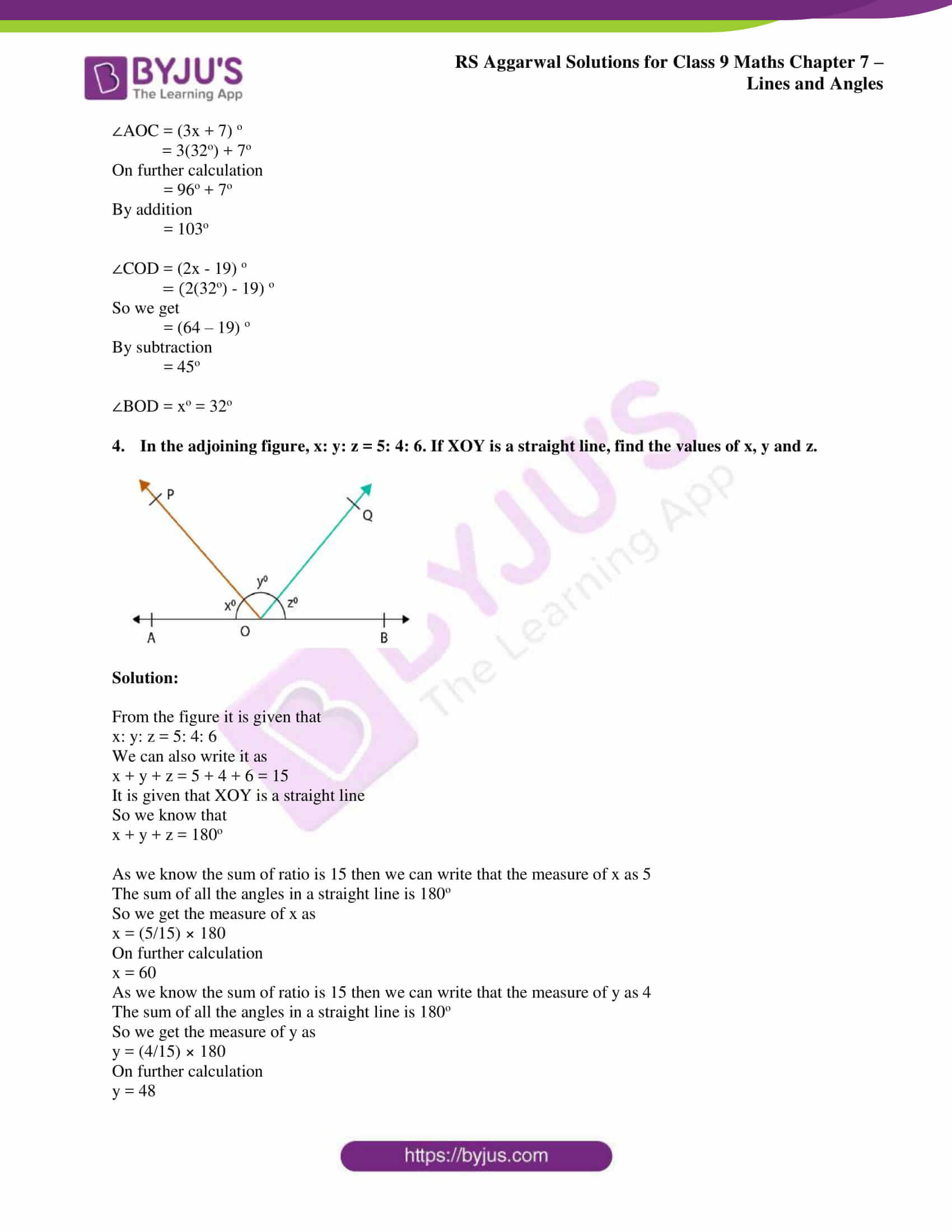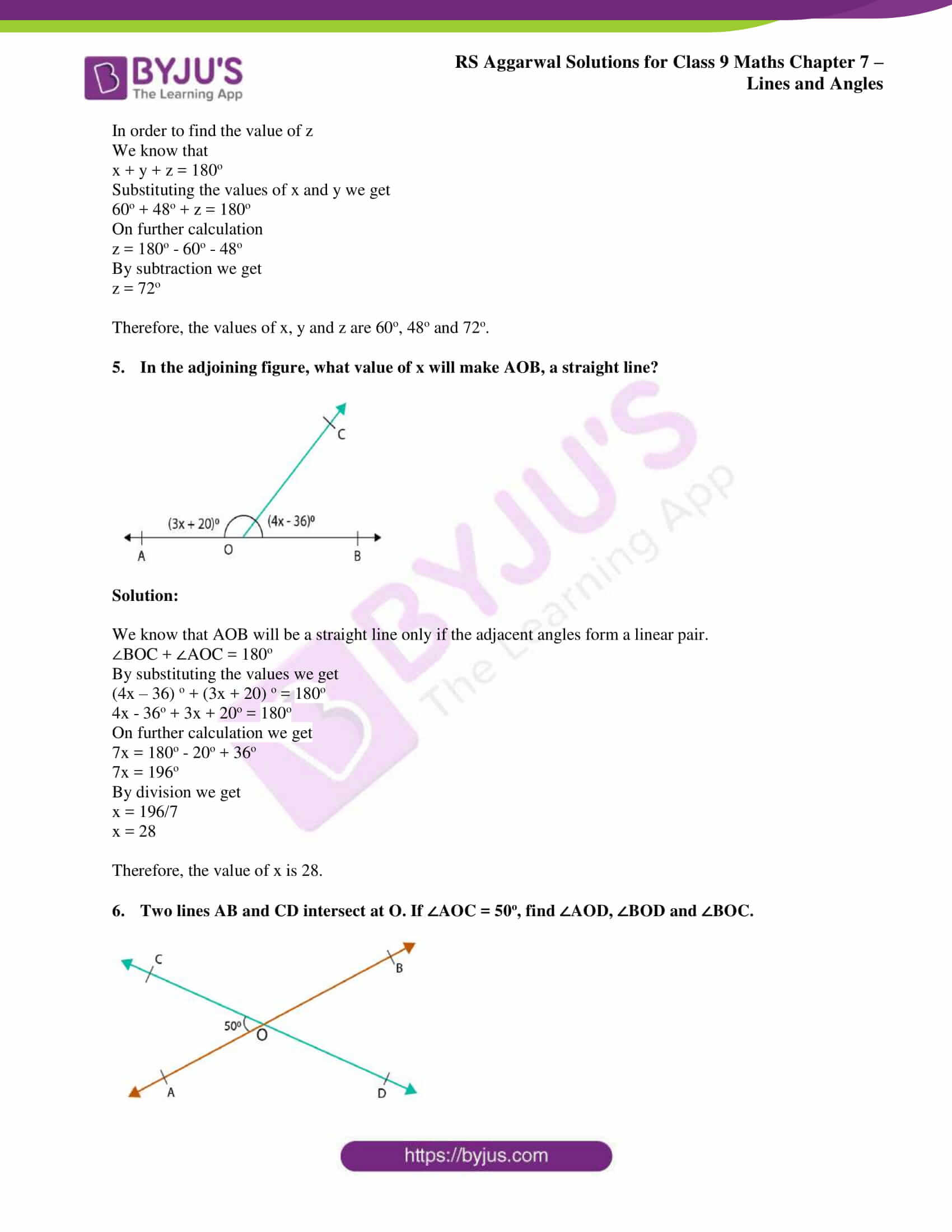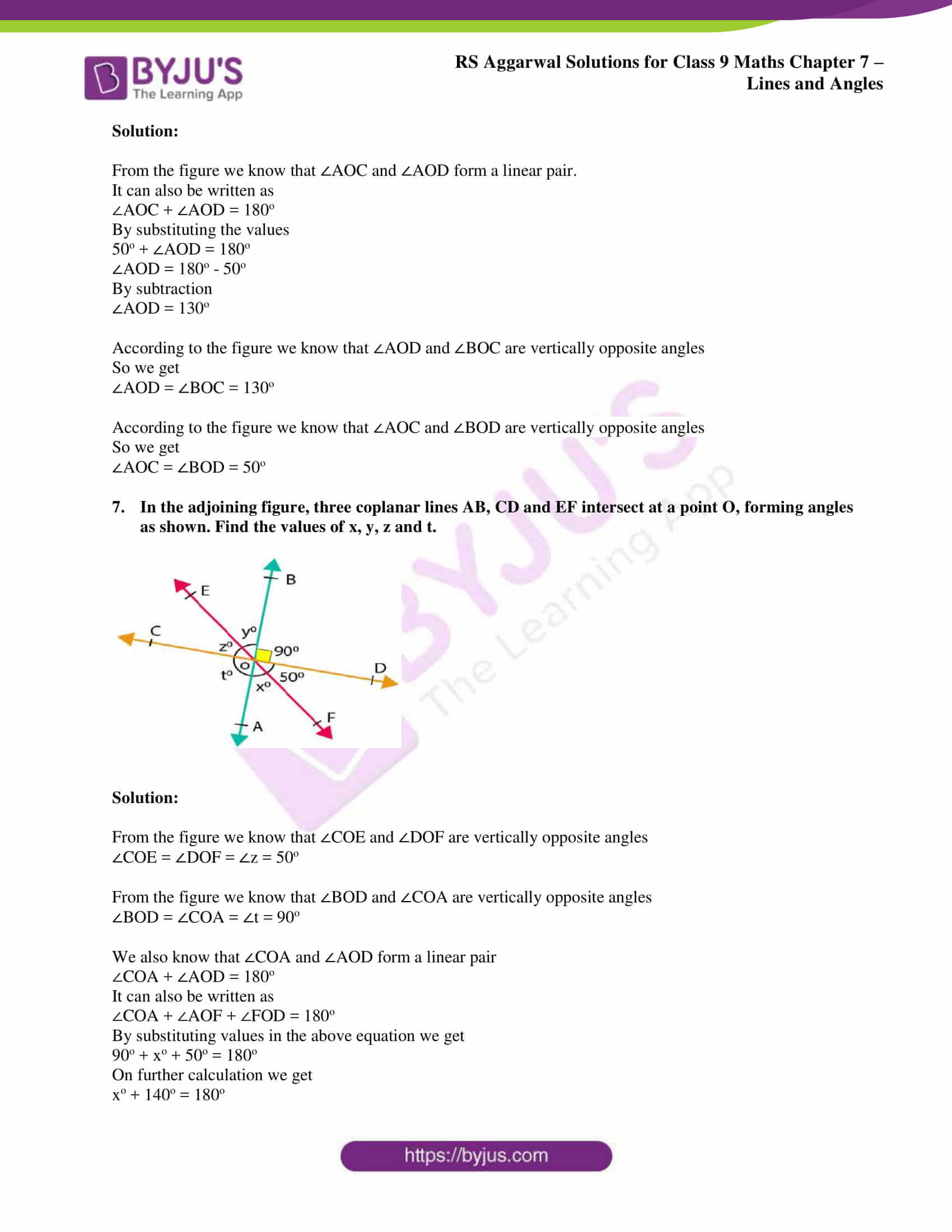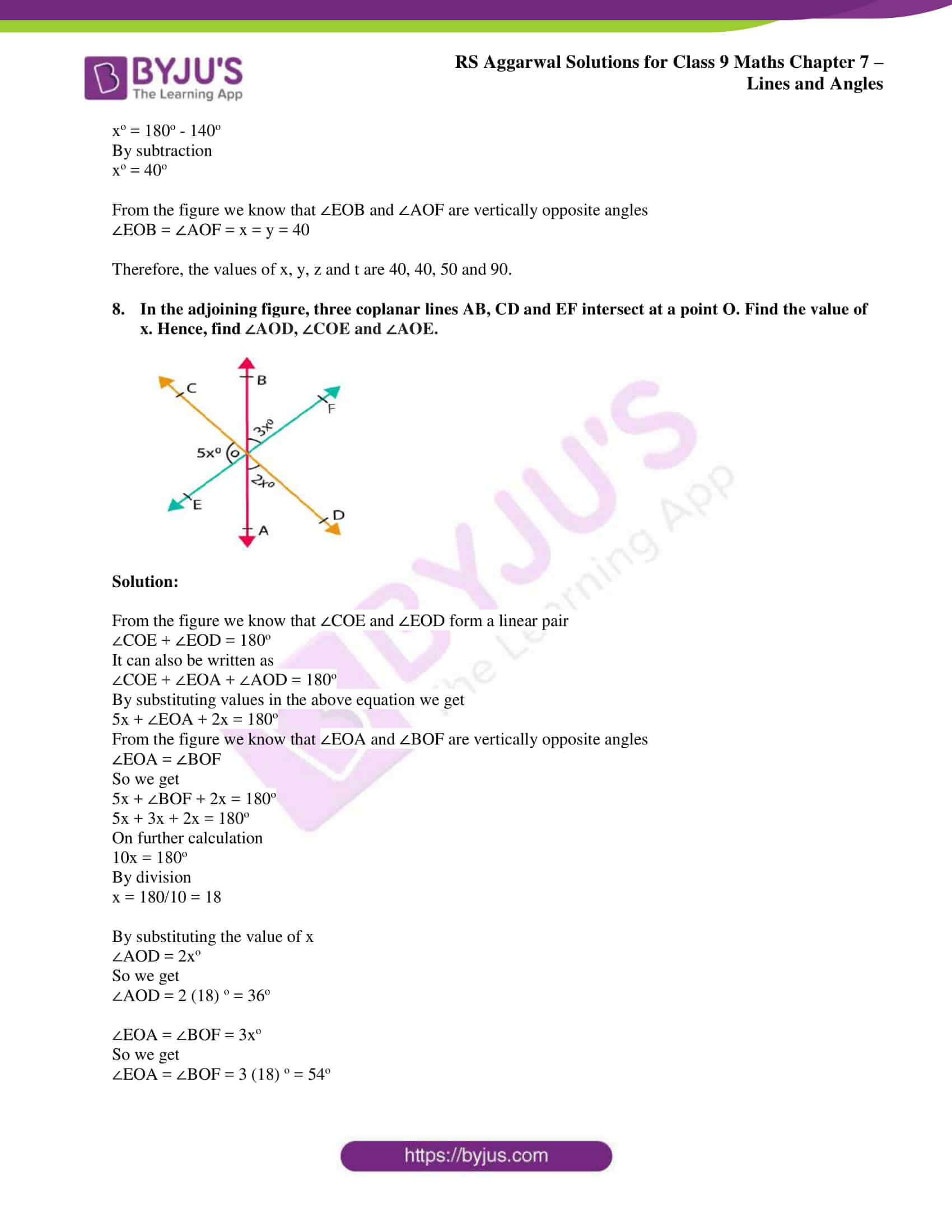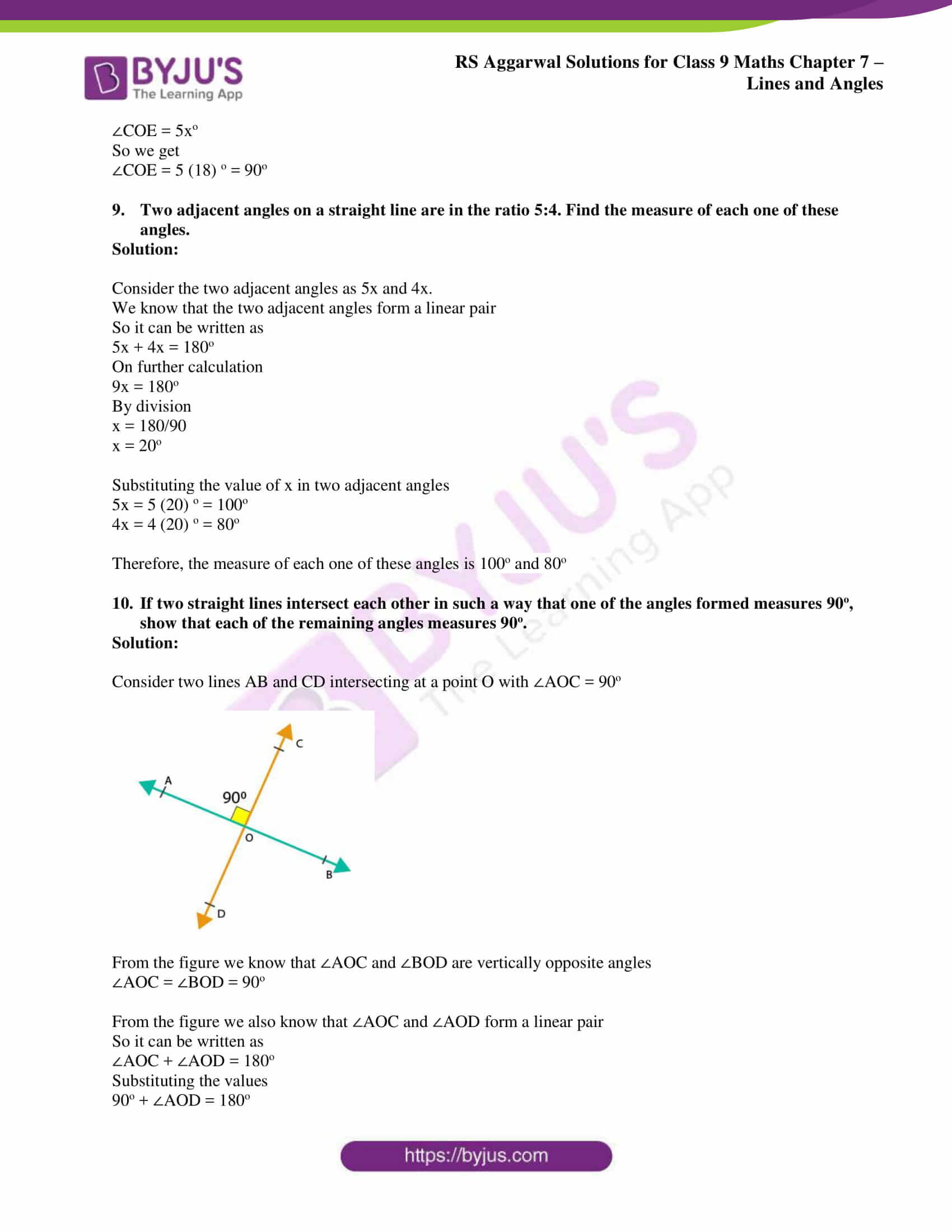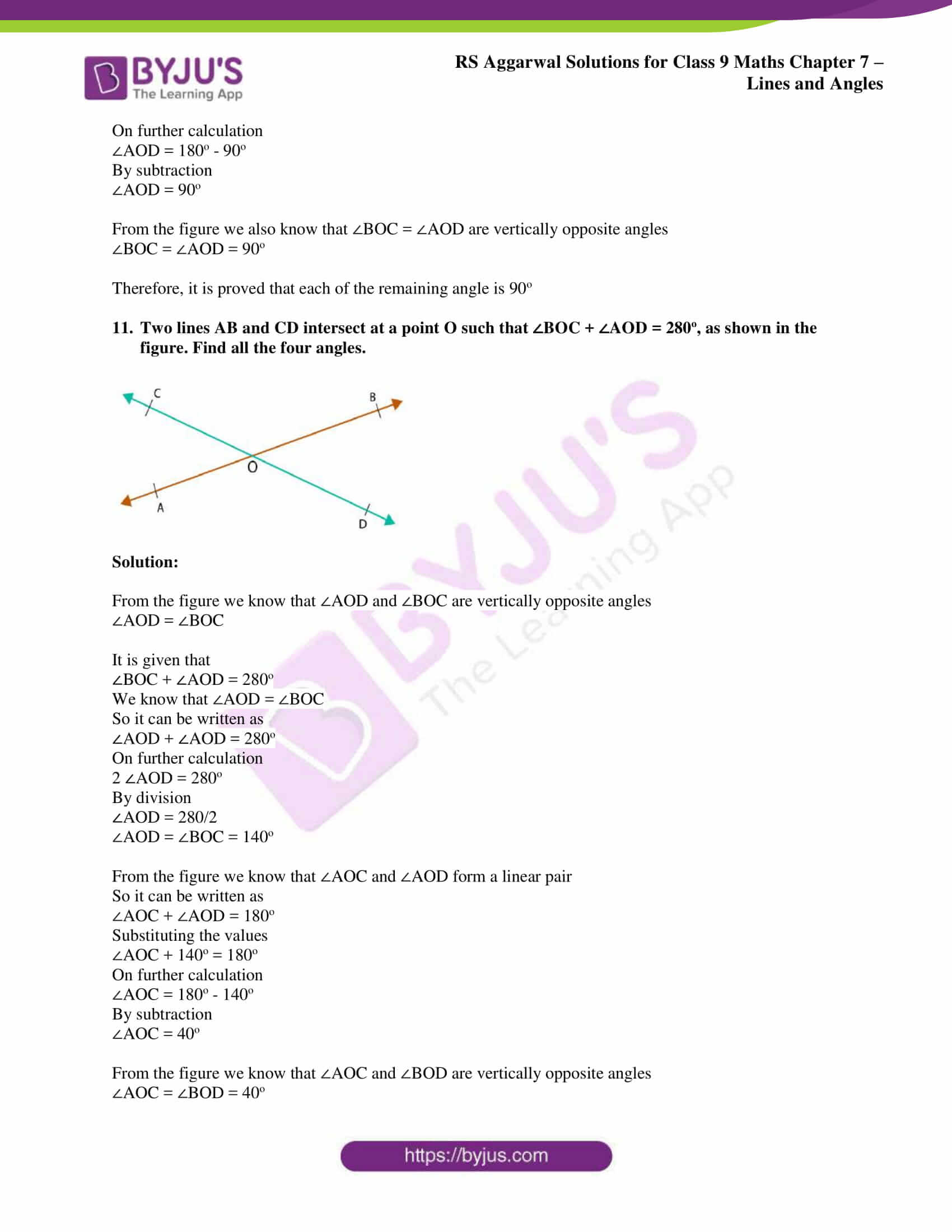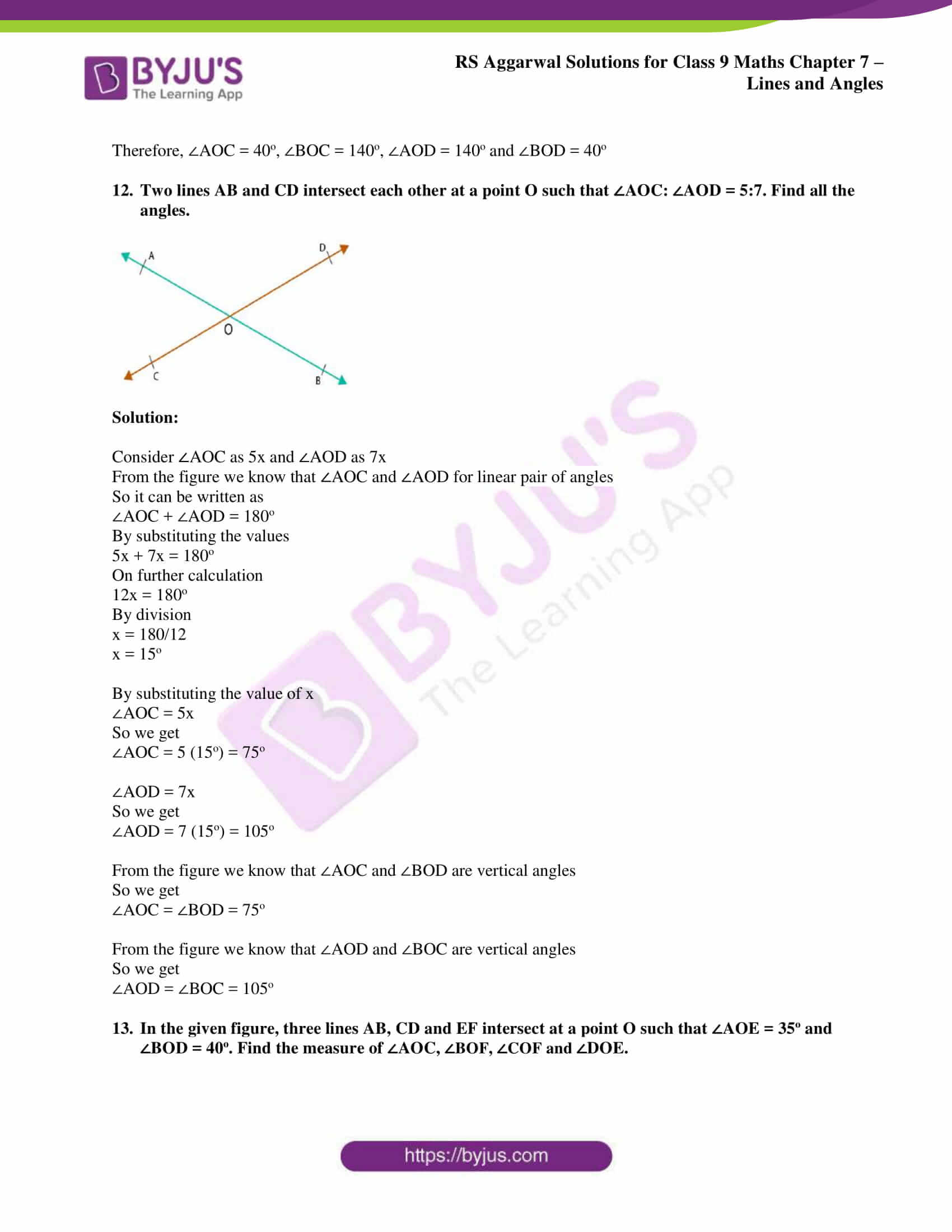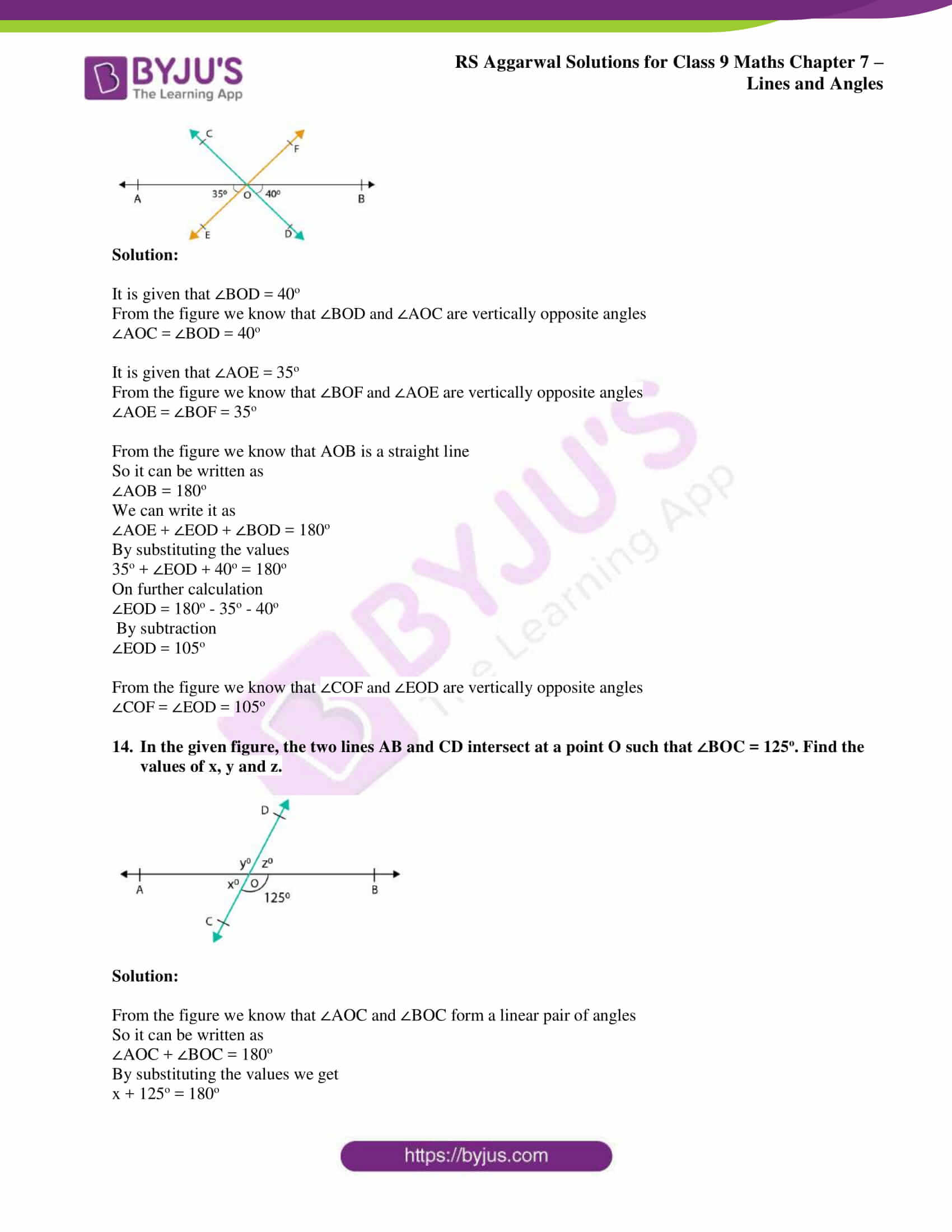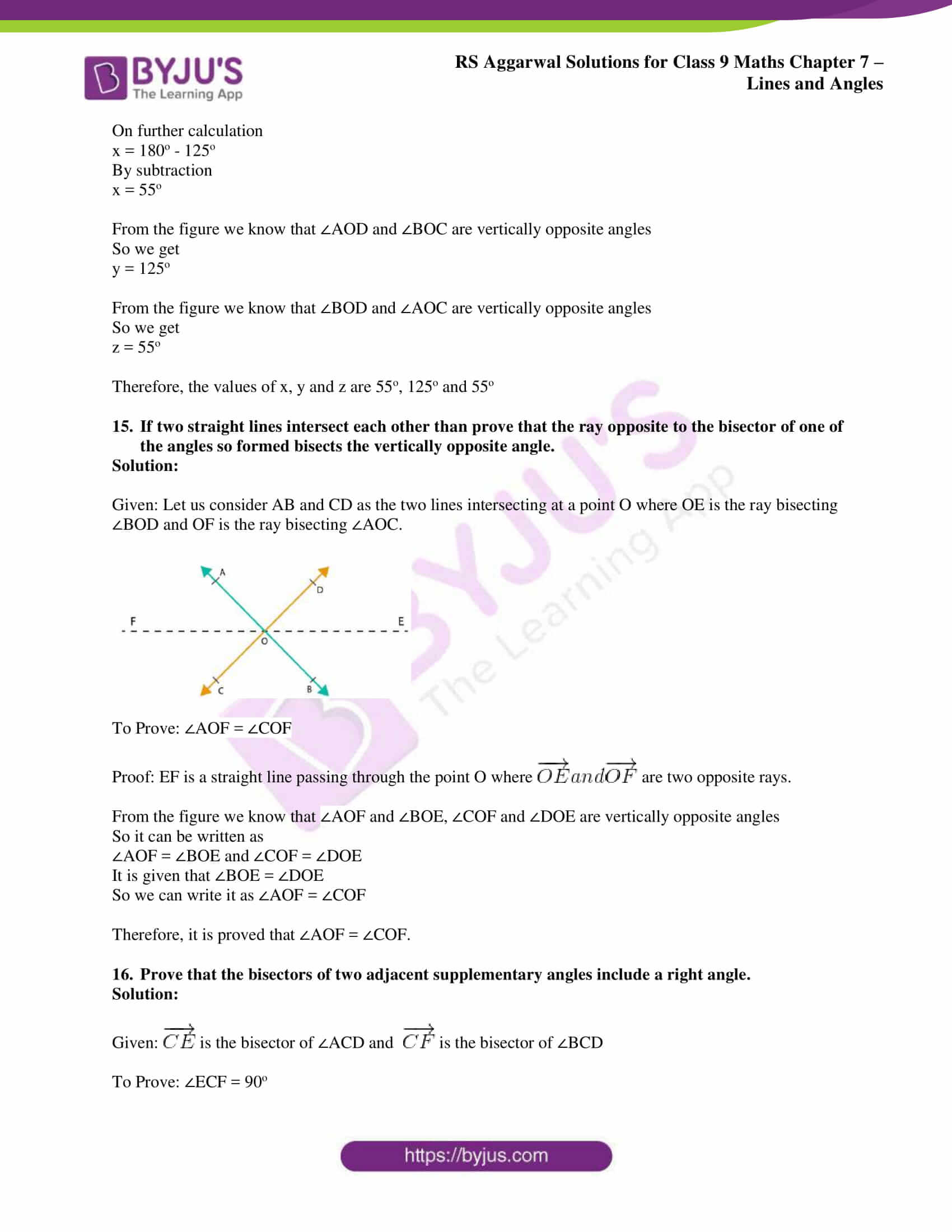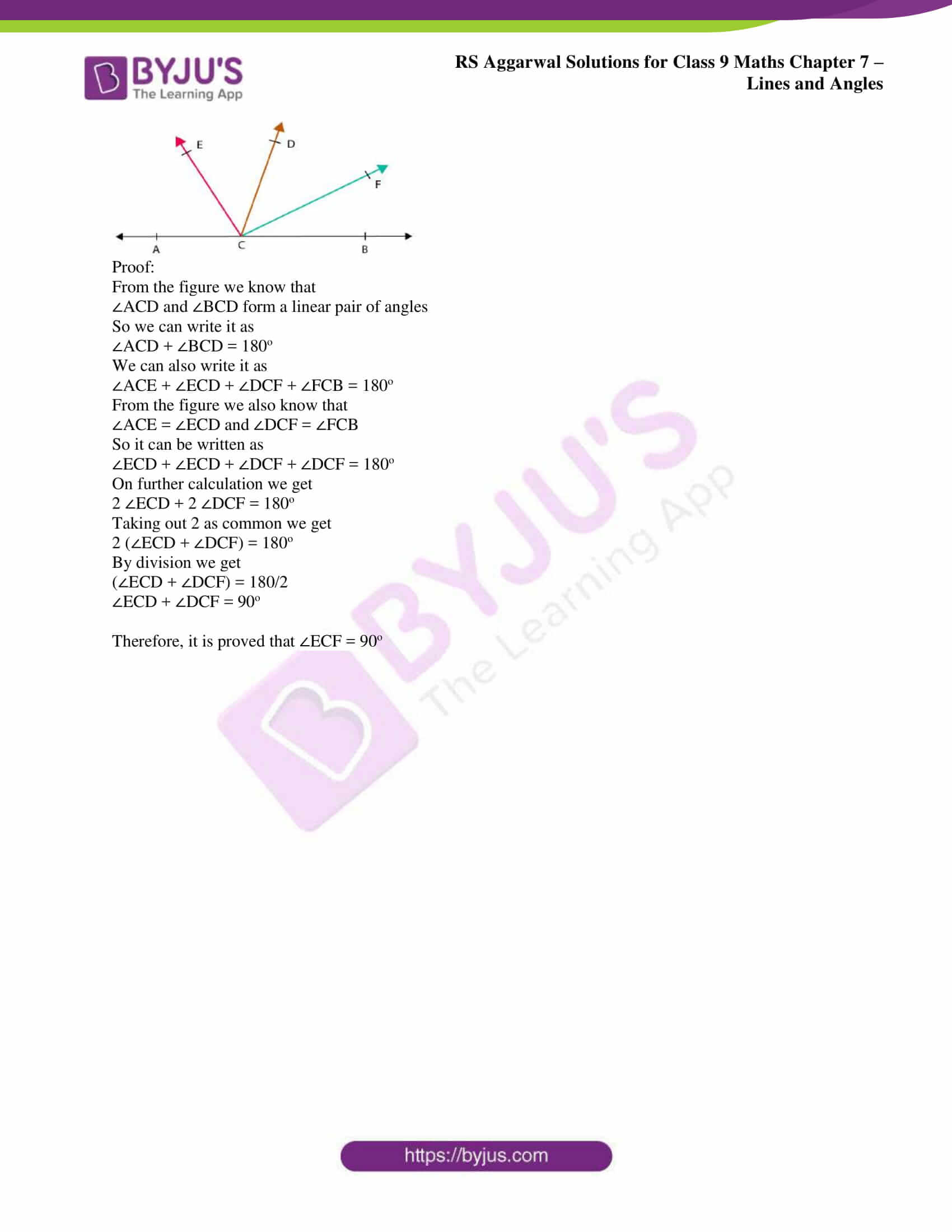## Access RS Aggarwal Solutions for Class 9 Chapter 7: Lines and Angles Exercise 7B

Exercise 7(B)

1. In the adjoining figure, AOB is a straight line. Find the value of x.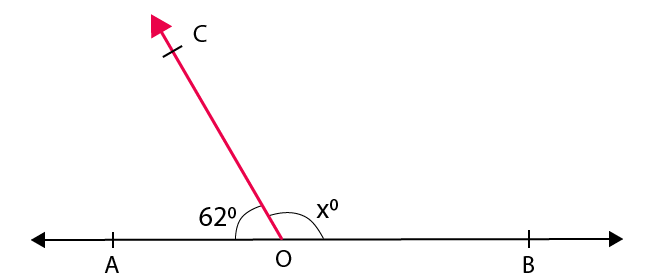Solution:

From the figure we know that ∠AOC and ∠BOC are a linear pair of angles

So we get

∠AOC + ∠BOC = 180o

We know that

62o + xo = 180o

On further calculation

xo = 180o – 62o

By subtraction

xo = 118o

Therefore, the value of x is 118.

2. In the adjoining figure, AOB is a straight line. Find the value of x. Hence, find ∠AOC and ∠BOD.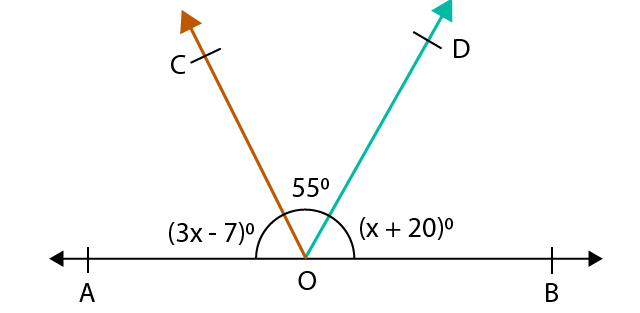Solution:

From the figure we know that ∠AOB is a straight line

So we get

∠AOB = 180o

It can also be written as

∠AOC + ∠COD + ∠BOD = 180o

By substituting the values

(3x – 7) o + 55o + (x + 20) o = 180o

3x – 7o + 55o + x + 20o = 180o

On further calculation

4x + 68o = 180o

4x = 112o

By division

x = 28o

By substituting the value of x we get

∠AOC = (3x – 7) o

= 3(28o) – 7o

On further calculation

= 84o – 7o

By subtraction

= 77o

∠BOD = (x + 20) o

= (28 + 20) o

= 48o

3. In the adjoining figure, AOB is a straight line. Find the value of x. Hence, find ∠AOC, ∠COD and ∠BOD.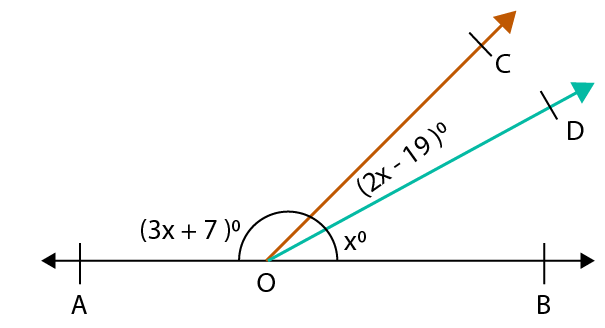Solution:

From the figure we know that ∠BOD and ∠AOD are a linear pair of angles

So we get

∠BOD + ∠AOD = 180o

It can also be written as

∠BOD + ∠COD + ∠COA = 180o

By substituting the values

xo + (2x – 19) o + (3x + 7) o = 180o

x + 2x – 19o + 3x + 7o = 180o

On further calculation

6x – 12o = 180o

6x = 180o + 12o

So we get

6x = 192o

By division

x = 32o

By substituting the value of x we get

∠AOC = (3x + 7) o

= 3(32o) + 7o

On further calculation

= 96o + 7o

= 103o

∠COD = (2x – 19) o

= (2(32o) – 19) o

So we get

= (64 – 19) o

By subtraction

= 45o

∠BOD = xo = 32o

4. In the adjoining figure, x: y: z = 5: 4: 6. If XOY is a straight line, find the values of x, y and z.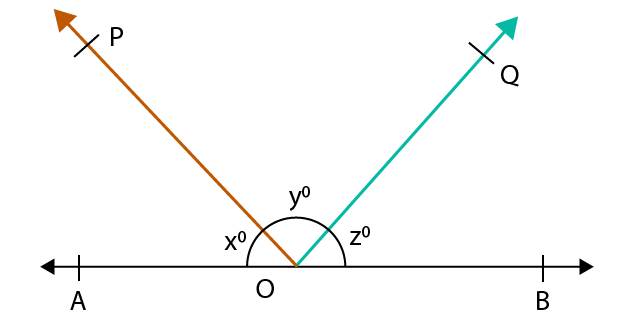Solution:

From the figure it is given that

x: y: z = 5: 4: 6

We can also write it as

x + y + z = 5 + 4 + 6 = 15

It is given that XOY is a straight line

So we know that

x + y + z = 180o

As we know the sum of ratio is 15 then we can write that the measure of x as 5

The sum of all the angles in a straight line is 180o

So we get the measure of x as

x = (5/15) × 180

On further calculation

x = 60

As we know the sum of ratio is 15 then we can write that the measure of y as 4

The sum of all the angles in a straight line is 180o

So we get the measure of y as

y = (4/15) × 180

On further calculation

y = 48

In order to find the value of z

We know that

x + y + z = 180o

Substituting the values of x and y we get

60o + 48o + z = 180o

On further calculation

z = 180o – 60o – 48o

By subtraction we get

z = 72o

Therefore, the values of x, y and z are 60o, 48o and 72o.

5. In the adjoining figure, what value of x will make AOB, a straight line?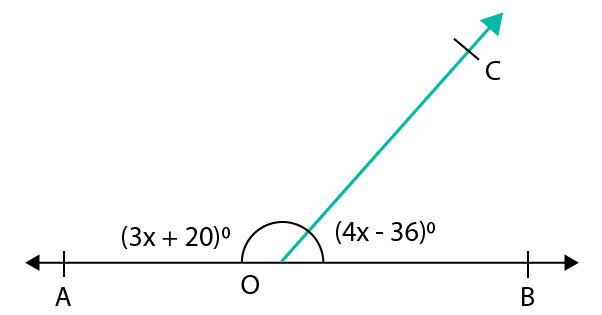Solution:

We know that AOB will be a straight line only if the adjacent angles form a linear pair.

∠BOC + ∠AOC = 180o

By substituting the values we get

(4x – 36) o + (3x + 20) o = 180o

4x – 36o + 3x + 20o = 180o

On further calculation we get

7x = 180o – 20o + 36o

7x = 196o

By division we get

x = 196/7

x = 28

Therefore, the value of x is 28.

6. Two lines AB and CD intersect at O. If ∠AOC = 50o, find ∠AOD, ∠BOD and ∠BOC.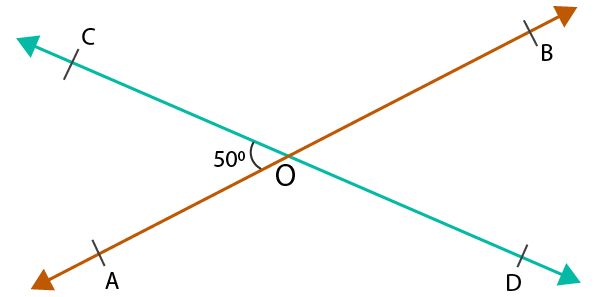Solution:

From the figure we know that ∠AOC and ∠AOD form a linear pair.

It can also be written as

∠AOC + ∠AOD = 180o

By substituting the values

50o + ∠AOD = 180o

∠AOD = 180o – 50o

By subtraction

∠AOD = 130o

According to the figure we know that ∠AOD and ∠BOC are vertically opposite angles

So we get

∠AOD = ∠BOC = 130o

According to the figure we know that ∠AOC and ∠BOD are vertically opposite angles

So we get

∠AOC = ∠BOD = 50o

7. In the adjoining figure, three coplanar lines AB, CD and EF intersect at a point O, forming angles as shown. Find the values of x, y, z and t.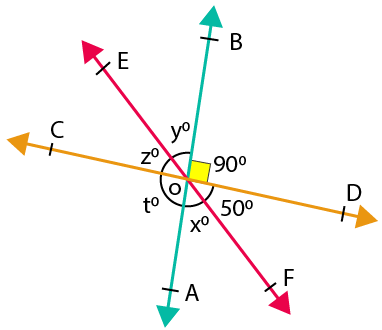Solution:

From the figure we know that ∠COE and ∠DOF are vertically opposite angles

∠COE = ∠DOF = ∠z = 50o

From the figure we know that ∠BOD and ∠COA are vertically opposite angles

∠BOD = ∠COA = ∠t = 90o

We also know that ∠COA and ∠AOD form a linear pair

∠COA + ∠AOD = 180o

It can also be written as

∠COA + ∠AOF + ∠FOD = 180o

By substituting values in the above equation we get

90o + xo + 50o = 180o

On further calculation we get

xo + 140o = 180o

xo = 180o – 140o

By subtraction

xo = 40o

From the figure we know that ∠EOB and ∠AOF are vertically opposite angles

∠EOB = ∠AOF = x = y = 40

Therefore, the values of x, y, z and t are 40, 40, 50 and 90.

8. In the adjoining figure, three coplanar lines AB, CD and EF intersect at a point O. Find the value of x. Hence, find ∠AOD, ∠COE and ∠AOE.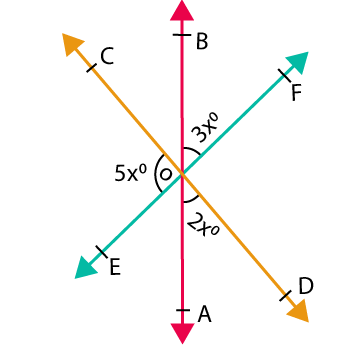Solution:

From the figure we know that ∠COE and ∠EOD form a linear pair

∠COE + ∠EOD = 180o

It can also be written as

∠COE + ∠EOA + ∠AOD = 180o

By substituting values in the above equation we get

5x + ∠EOA + 2x = 180o

From the figure we know that ∠EOA and ∠BOF are vertically opposite angles

∠EOA = ∠BOF

So we get

5x + ∠BOF + 2x = 180o

5x + 3x + 2x = 180o

On further calculation

10x = 180o

By division

x = 180/10 = 18

By substituting the value of x

∠AOD = 2xo

So we get

∠AOD = 2 (18) o = 36o

∠EOA = ∠BOF = 3xo

So we get

∠EOA = ∠BOF = 3 (18) o = 54o

∠COE = 5xo

So we get

∠COE = 5 (18) o = 90o

9. Two adjacent angles on a straight line are in the ratio 5:4. Find the measure of each one of these angles.

Solution:

Consider the two adjacent angles as 5x and 4x.

We know that the two adjacent angles form a linear pair

So it can be written as

5x + 4x = 180o

On further calculation

9x = 180o

By division

x = 180/90

x = 20o

Substituting the value of x in two adjacent angles

5x = 5 (20) o = 100o

4x = 4 (20) o = 80o

Therefore, the measure of each one of these angles is 100o and 80o

10. If two straight lines intersect each other in such a way that one of the angles formed measures 90o, show that each of the remaining angles measures 90o.

Solution:

Consider two lines AB and CD intersecting at a point O with ∠AOC = 90o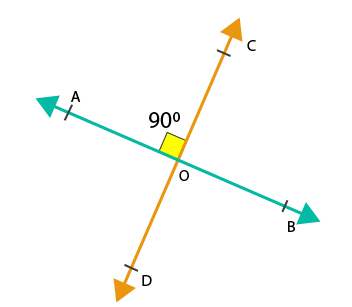From the figure we know that ∠AOC and ∠BOD are vertically opposite angles

∠AOC = ∠BOD = 90o

From the figure we also know that ∠AOC and ∠AOD form a linear pair

So it can be written as

∠AOC + ∠AOD = 180o

Substituting the values

90o + ∠AOD = 180o

On further calculation

∠AOD = 180o – 90o

By subtraction

∠AOD = 90o

From the figure we also know that ∠BOC = ∠AOD are vertically opposite angles

∠BOC = ∠AOD = 90o

Therefore, it is proved that each of the remaining angle is 90o

11. Two lines AB and CD intersect at a point O such that ∠BOC + ∠AOD = 280o, as shown in the figure. Find all the four angles.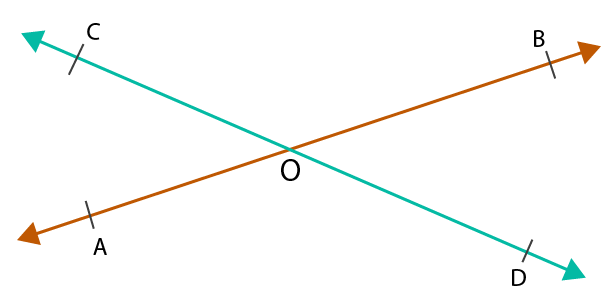Solution:

From the figure we know that ∠AOD and ∠BOC are vertically opposite angles

∠AOD = ∠BOC

It is given that

∠BOC + ∠AOD = 280o

We know that ∠AOD = ∠BOC

So it can be written as

∠AOD + ∠AOD = 280o

On further calculation

2 ∠AOD = 280o

By division

∠AOD = 280/2

∠AOD = ∠BOC = 140o

From the figure we know that ∠AOC and ∠AOD form a linear pair

So it can be written as

∠AOC + ∠AOD = 180o

Substituting the values

∠AOC + 140o = 180o

On further calculation

∠AOC = 180o – 140o

By subtraction

∠AOC = 40o

From the figure we know that ∠AOC and ∠BOD are vertically opposite angles

∠AOC = ∠BOD = 40o

Therefore, ∠AOC = 40o, ∠BOC = 140o, ∠AOD = 140o and ∠BOD = 40o

12. Two lines AB and CD intersect each other at a point O such that ∠AOC: ∠AOD = 5:7. Find all the angles.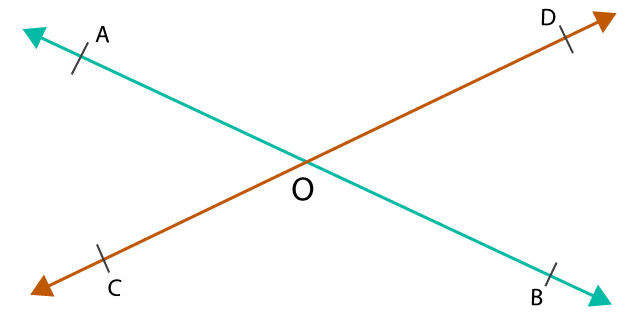Solution:

Consider ∠AOC as 5x and ∠AOD as 7x

From the figure we know that ∠AOC and ∠AOD for linear pair of angles

So it can be written as

∠AOC + ∠AOD = 180o

By substituting the values

5x + 7x = 180o

On further calculation

12x = 180o

By division

x = 180/12

x = 15o

By substituting the value of x

∠AOC = 5x

So we get

∠AOC = 5 (15o) = 75o

∠AOD = 7x

So we get

∠AOD = 7 (15o) = 105o

From the figure we know that ∠AOC and ∠BOD are vertical angles

So we get

∠AOC = ∠BOD = 75o

From the figure we know that ∠AOD and ∠BOC are vertical angles

So we get

∠AOD = ∠BOC = 105o

13. In the given figure, three lines AB, CD and EF intersect at a point O such that ∠AOE = 35o and ∠BOD = 40o. Find the measure of ∠AOC, ∠BOF, ∠COF and ∠DOE.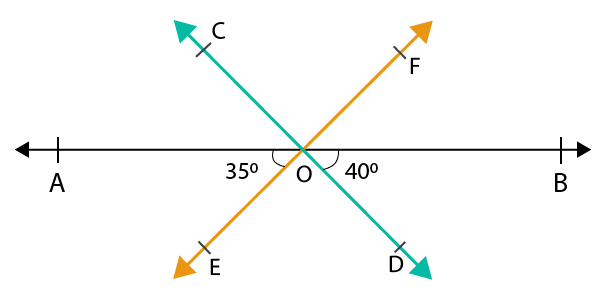Solution:

It is given that ∠BOD = 40o

From the figure we know that ∠BOD and ∠AOC are vertically opposite angles

∠AOC = ∠BOD = 40o

It is given that ∠AOE = 35o

From the figure we know that ∠BOF and ∠AOE are vertically opposite angles

∠AOE = ∠BOF = 35o

From the figure we know that AOB is a straight line

So it can be written as

∠AOB = 180o

We can write it as

∠AOE + ∠EOD + ∠BOD = 180o

By substituting the values

35o + ∠EOD + 40o = 180o

On further calculation

∠EOD = 180o – 35o – 40o

By subtraction

∠EOD = 105o

From the figure we know that ∠COF and ∠EOD are vertically opposite angles

∠COF = ∠EOD = 105o

14. In the given figure, the two lines AB and CD intersect at a point O such that ∠BOC = 125o. Find the values of x, y and z.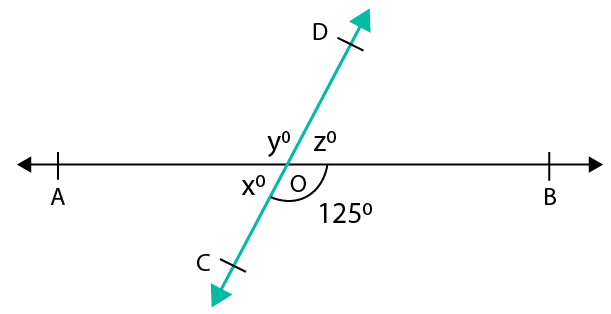Solution:

From the figure we know that ∠AOC and ∠BOC form a linear pair of angles

So it can be written as

∠AOC + ∠BOC = 180o

By substituting the values we get

x + 125o = 180o

On further calculation

x = 180o – 125o

By subtraction

x = 55o

From the figure we know that ∠AOD and ∠BOC are vertically opposite angles

So we get

y = 125o

From the figure we know that ∠BOD and ∠AOC are vertically opposite angles

So we get

z = 55o

Therefore, the values of x, y and z are 55o, 125o and 55o

15. If two straight lines intersect each other than prove that the ray opposite to the bisector of one of the angles so formed bisects the vertically opposite angle.

Solution:

Given: Let us consider AB and CD as the two lines intersecting at a point O where OE is the ray bisecting ∠BOD and OF is the ray bisecting ∠AOC.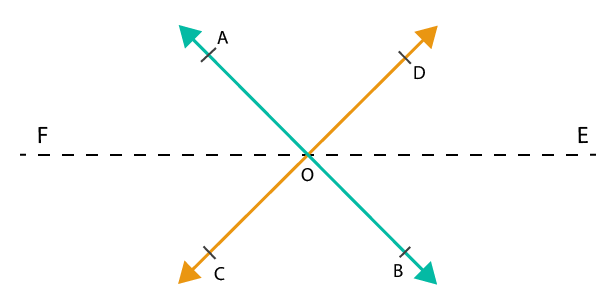To Prove: ∠AOF = ∠COF

Proof: EF is a straight line passing through the point O where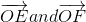are two opposite rays.

From the figure we know that ∠AOF and ∠BOE, ∠COF and ∠DOE are vertically opposite angles

So it can be written as

∠AOF = ∠BOE and ∠COF = ∠DOE

It is given that ∠BOE = ∠DOE

So we can write it as ∠AOF = ∠COF

Therefore, it is proved that ∠AOF = ∠COF.

16. Prove that the bisectors of two adjacent supplementary angles include a right angle.

Solution:

Given: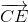is the bisector of ∠ACD and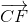is the bisector of ∠BCD

To Prove: ∠ECF = 90o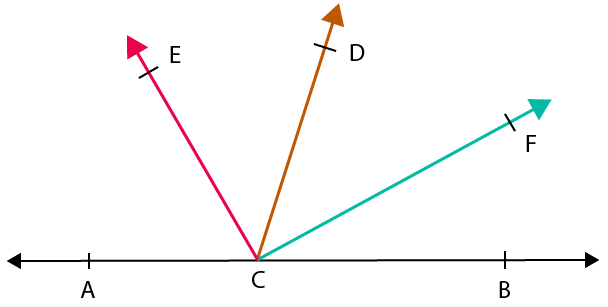Proof:

From the figure we know that

∠ACD and ∠BCD form a linear pair of angles

So we can write it as

∠ACD + ∠BCD = 180o

We can also write it as

∠ACE + ∠ECD + ∠DCF + ∠FCB = 180o

From the figure we also know that

∠ACE = ∠ECD and ∠DCF = ∠FCB

So it can be written as

∠ECD + ∠ECD + ∠DCF + ∠DCF = 180o

On further calculation we get

2 ∠ECD + 2 ∠DCF = 180o

Taking out 2 as common we get

2 (∠ECD + ∠DCF) = 180o

By division we get

(∠ECD + ∠DCF) = 180/2

∠ECD + ∠DCF = 90o

Therefore, it is proved that ∠ECF = 90o

### RS Aggarwal Solutions Class 9 Maths Chapter 7 – Lines and Angles Exercise 7B

RS Aggarwal Solutions Class 9 Maths Chapter 7 Lines and Angles Exercise 7B is the second exercise which has solutions on finding adjacent angles, linear pair of angles and vertically opposite angles.

### Key features of RS Aggarwal Solutions for Class 9 Maths Chapter 7: Lines and Angles Exercise 7B

• Here the students mainly learn about angles, corollaries and theorems based on Lines and Angles.
• Revising the concepts of the chapter 7 is made easy with the help of solutions prepared by our faculty.
• PDF format of solutions can be downloaded by the students for free which is a vital resource to perform well in the exams.
• The solutions are prepared keeping the mind the CBSE exam pattern and weightage for the concepts.